## Java 中 HashMap 的工作机制

1、一句话回答
2、什么是哈希
3、关于Entry类的一点介绍
4、put()方法实际上做了什么
5、get()方法内部工作机制
6、注意点

```static class Entry<K,V> implements Map.Entry<K,V>
{
final K key;
V value;
Entry<K,V> next;
final int hash;
...//More code goes here
}```

put()方法实际上做了什么

```/**
* The table, resized as necessary. Length MUST Always be a power of two.
*/
transient Entry[] table;```

```/**
* Associates the specified value with the specified key in this map.
* If the map previously contained a mapping for the key, the old
* value is replaced.
*
* @param key key with which the specified value is to be associated
* @param value value to be associated with the specified key
* @return the previous value associated with <tt>key</tt>, or
*&nbsp;&nbsp;&nbsp;&nbsp;&nbsp;&nbsp;&nbsp;&nbsp; <tt>null</tt> if there was no mapping for <tt>key</tt>.
*&nbsp;&nbsp;&nbsp;&nbsp;&nbsp;&nbsp;&nbsp;&nbsp; (A <tt>null</tt> return can also indicate that the map
*&nbsp;&nbsp;&nbsp;&nbsp;&nbsp;&nbsp;&nbsp;&nbsp; previously associated <tt>null</tt> with <tt>key</tt>.)
*/
public V put(K key, V value) {
if (key == null)
return putForNullKey(value);
int hash = hash(key.hashCode());
int i = indexFor(hash, table.length);
for (Entry<K,V> e = table[i]; e != null; e = e.next) {
Object k;
if (e.hash == hash && ((k = e.key) == key || key.equals(k))) {
V oldValue = e.value;
e.value = value;
e.recordAccess(this);
return oldValue;
}
}

modCount++;
return null;
}```

get方法的工作机制

```/**
* Returns the value to which the specified key is mapped,
* or {@code null} if this map contains no mapping for the key.
*
* <p>More formally, if this map contains a mapping from a key
* {@code k} to a value {@code v} such that {@code (key==null ? k==null :
* key.equals(k))}, then this method returns {@code v}; otherwise
* it returns {@code null}.&nbsp; (There can be at most one such mapping.)
*
* <p>A return value of {@code null} does not <i>necessarily</i>
* indicate that the map contains no mapping for the key; it's also
* possible that the map explicitly maps the key to {@code null}.
* The {@link #containsKey containsKey} operation may be used to
* distinguish these two cases.
*
* @see #put(Object, Object)
*/
public V get(Object key) {
if (key == null)
return getForNullKey();
int hash = hash(key.hashCode());
for (Entry<K,V> e = table[indexFor(hash, table.length)];
e != null;
e = e.next) {
Object k;
if (e.hash == hash && ((k = e.key) == key || key.equals(k)))
return e.value;
}
return null;
}```

• 存储Entry对象的数据结构是一个叫做Entry类型的table数组。
• Key对象的hashCode()需要用来计算Entry对象的存储位置。
• Key对象的equals()方法需要用来维持Map中对象的唯一性。
• get()和put()方法跟Value对象的hashCode和equals方法无关。
• null的hashCode总是0，这样的Entry对象总是被存储在数组的第一个位置

00
f
“get()和put()方法跟Value对象的hashCode和equals方法无关"  这个表示质疑！
f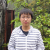0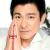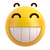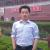0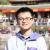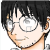00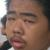#### 引用来自“OrionBox”的答案

0Map中的equals和hashCode